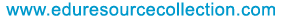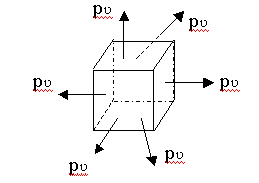@import url(http://www.google.com/cse/api/branding.css);Custom Search The content of the site is exclusively owned byHome | School Level | School Projects | Examinations | Civil | Food Processing | Seminars | Software Testing | Warehousing & Inventory | Networking

# Strength of Materials - Relationship among the elastic constants

8.1. Relationship between modulus of elasticity and modulus of rigidity

Consider a square element ABCD of side ‘a’ subjected to simple shear of intensity q as shown in figure.
It is deformed to the shape AB’C’D under the shear stress. Drop perpendicular BE to the diagonal DB’. Let ? be the shear strain induced and let N be the modulus of rigidity.
The diagonal DB gets elongated to DB’. Hence there is tensile strain et in the diagonal.
et = (DB’ – DB) / DB = EB’ / DB
since this deformation is very small we can take L BB’E = 45º
EB’ = BB’ / v2 = AB tan ? / v2 = a tan ? / v2
DB = v2 a
et = (a tan ? / v2)/ v2 a = tan ? /2 = = ? / 2 since ? is small
ie et = ½ X q/N ------------------ (1)
We know that stress along the diagonal DB is a pure tensile stress pt = q and that along the diagonal AC is a pure compressive stress pc also equal to q. hence the strain along the diagonal DB is et = q/E + 1/m X q/E
Ie et = q/E (1+1/m) ------------------ (2)
From (1) and (2) we have,
E = 2N(1+1/m)
This is the required relationship between E and N.

8.2. Relationship between Modulus of Elasticity E and Bulk Modulus KConsider a cube element subjected to volumetric tensile stress p? in X,Y and Z directions. Stress in each direction is equal to p?. ie px = py = pz =p?
Consider strains induced in X-direction by these stresses. px induces tensile strain , while py and pz induces compressive strains. Therefore,
ex = px/E – 1/m[py/E + pz/E] = p?/E[1-2/m]
due to the perfect symmetry in geometry and stresses
ey = p?/E[1-2/m]
ez = p?/E[1-2/m]
K = p ? / e ? =p ?/(ex+ey+ez) = p?/[3p?/E(1-2/m)]
ie E = 3K(1-2/m ) is the required relationship.

8.3. Relationship among the constants

From above,
E = 2N[1+(1/m)] and E = 3K[1-(2/m )]
E = 3K[1-2(E/2N -1)] = 3K[1-E/N +2]
9K = E[1+(3K/N)] = E[(N+3K)/N]
E = 9NK/(N+3K)

9. Bars of uniform section

Consider a bar of length l and Cross sectional area A. Let P be the axial pull on the bar,p the stresss induced ,e the strain in the bar and dl is the elongation.
Then p= P/A
e= p/E =P/(AE) -------------(1)
e= dl/l -------------(2)
equating (1) and (2)
dl = Pl / (AE)